﻿ OceanInfo平台中舰船操纵运动控制研究
 舰船科学技术2016, Vol. 38Issue (6): 63-66PDF
OceanInfo平台中舰船操纵运动控制研究

Research on ship steering control in OceanInfo platform
HUANG Qian, XU Xiao-gang, LI Tian-wei, YI Cheng-tao, JIN Xin
Abstract: Against the ship steering control in OceanInfo platform, the chaotic motion and its control in the process of ship course keeping are discussed. The PID control method is used to control the chaotic ship steering and course keeping based on its simplicity and validity, but the control parameters are difficult to be tuned, so an improved dynamic chaos particle swarm optimization algorithm for the PID controller parameters tuning is proposed and the simulation experiments are carried. The results show that, the PID controller parameters tuned by the proposed optimization algorithm can realize ship course keeping and chaotic motion control more effectively, and the proposed algorithm is easy to program.
Key words: OceanInfo platform     course control     chaotic motion     PID control
0 引言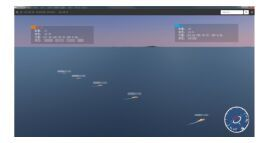图 1 模拟海空演习场景 Fig. 1 Scene of military manoeuvre simulation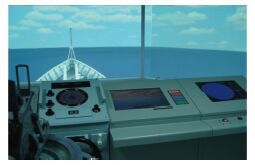图 2 舰船操纵运动模拟 Fig. 2 Ship steering simulation

1 OceanInfo 平台舰船操纵运动模型

 $\left\{ {\begin{array}{*{20}{l}} {{{\dot x}_1} = {x_2},}\\ {{{\dot x}_2} =-(a + e \cdot {K_p}){x_2}-(b + d \cdot {K_p}){x_1}-cx_1^3}+\\ {\;\;\;\;\; d \cdot {K_p}B\sin (\omega t) + e \cdot {K_p}B\omega \cos (\omega t) \text{。}} \end{array}} \right.$ (1)

2 航向保持中的混沌问题

 $\left\{ {\begin{array}{*{20}{l}} {{{\dot x}_1} = {x_2},}\\ {{{\dot x}_2} =-0.320 \,8{x_2} + 0.2715{x_1}-4.394 \,5x_1^3}+\\ {\;\;\;\;\; 0.103 \,68\cos (0.8t) + 0.078 \,8\sin (0.8t) \text{。}} \end{array}} \right.$ (2)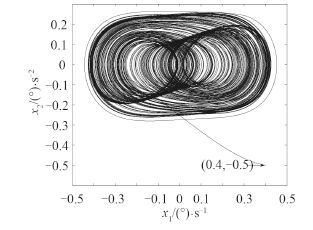图 3 系统相位图 Fig. 3 Figure of system phase

3 PID 参数改进混沌粒子群优化整定 3.1 改进混沌粒子群优化算法

 ${\omega _{(t + 1) }} = {\omega _{\min }} + \frac{{({\omega _{\max }}-{\omega _{\min }})}}{{{e^{(t \times \frac{{{f_{{\rm{t}}\_{\rm{avg}}}}}}{{{f_{\max }}-{f_{\min }}}})}}}} \text{。}$ (3)

Lorenz 系统是最早得到研究的混沌动力学系统，考察 Lorenz 方程，发现该系统恰好有 3 个状态变量 x，y，z，可对应为种群粒子位置的三维向量（即为 PID 控制器的 3 个参数），同时由于系统具有明显的混沌特征，本文选择将该系统引入优化算法，用于种群粒子在陷入局部最优后获得继续搜索的能力。当优化算法达到设定的条件（即种群粒子连续 k 次适应度值没有改善）时，产生一个三维随机向量初始值 ${d_0} = {[{d_{x0}},{d_{y0}},{d_{z0}}]^{\rm{T}}}$，对应 Lorenz 系统中的 3 个状态变量，根据 Lorenz 方程开始混沌序列迭代，利用迭代结果对符合条件的种群粒子进行动态混沌扰动，并进行种群粒子适应度值的改善情况判断以决定后续算法，其基本步骤如下：

1）初始化。设定混沌迭代步数 M，迭代步长 $\Delta s$，给初始值 d0 赋值，记录混沌扰动前种群粒子的适应度值 ${f_{bef}}$

2）混沌扰动。根据 M$\Delta s$，依据式（4）产生混沌序列，进行混沌迭代，得到状态变量 ${d_M} = {[{x_{xM}},{x_{yM}},{x_{zM}}]^{\rm{T}}}$，对种群粒子进行位置改变，并计算扰动后种群粒子的适应度值 ${f_{aft}}$

3）扰动结果判断。若 ${f_{aft}} < {f_{bef}}$，说明种群粒子适应度值得到改善，则更新粒子位置，进入改进 PSO 算法；若 faftfbef，说明混沌扰动没有效果，则转向步骤2，继续进行混沌扰动。

3.3 PID 参数优化算法流程

1）种群初始化。在可行解空间内初始化种群的规模 N，种群粒子的初始位置、速度，种群中每一粒子为 PID 控制器参数构成的三维向量 x0 = [x01x02x03]T，设置最大演化步数 n 等参数，对种群粒子的位置、速度进行范围限制。

2）适应度值计算。根据 OceanInfo 平台采用的舰船操纵运动模型，计算种群粒子的适应值，确定种群的当前局部最优位置 pi 和全局最优位置 pg。选择 ITSE 指标作为适应度函数：

 $ITSEF:itness{\rm{ = }}\int_0^{ + \infty } {{t^2}{e^2}\left( t \right){\rm{d}}t} \text{。}$ (4)

3）种群粒子更新。依据本文的改进 PSO 算法对种群粒子位置、速度、种群局部最优位置 pi 和全局最优位置 pg 进行更新，判断粒子是否陷入局部最优，采用如下的标准：

 ${\Delta _{fi}} = \frac{{|{f_i}-{f_{pbesti}}|}}{{{f_{pbesti}}}},(i = 1,2,\cdots ,n) \text{。}$ (5)

4）终止条件判断。若优化算法满足所设定的终止条件（达到最大演化步数或受控混沌系统输出达到设计指标），停止种群演化，输出全局最优位置；反之，返回步骤2。

4 舰船混沌运动的 PID 控制研究 4.1 PID 控制器设计

 \left\{ \begin{aligned} \,{{\dot x}_1} = & {x_2},\\ \,{{\dot x}_2} = &-0.3208{x_2} + 0.2715{x_1}-4.3945x_1^3 + \\ & 0.10368\cos (0.8t) + 0.0788\sin (0.8t) + u(t),\\ \!\!\!\! u(t) & = {K_p}e(t) + {K_i}\int {e(t){\rm{d}}t + {K_d}\frac{{{\rm{d}}e(t)}}{{{\rm{d}}t}}} \text{。} \end{aligned} \right. (6)

4.2 PID 控制仿真实验与分析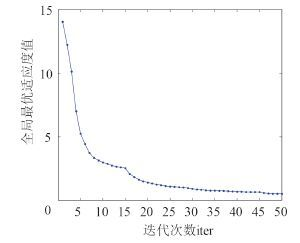图 4 种群适应度值曲线 Fig. 4 Curve of fitness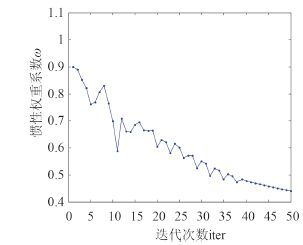图 5 惯性权重系数 ω 曲线 Fig. 5 Curve of inertial weight ω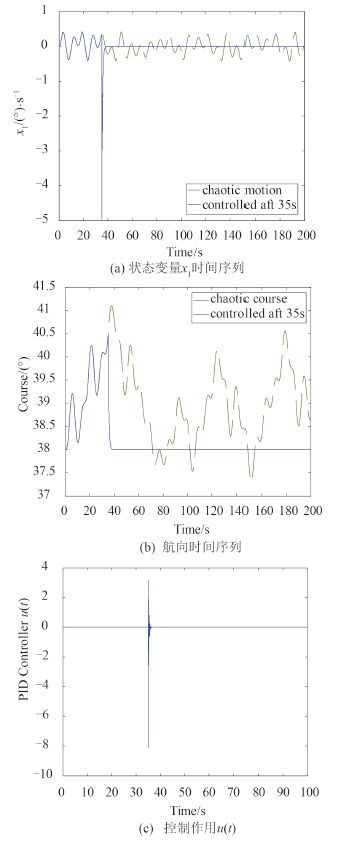图 6 本文改进算法优化的PID控制过程 Fig. 6 Process of PID control optimized by our algorithm
5 结语

  李耀阳, 郭涛, 刘敬明. 浅议虚拟技术在海军指挥信息系统中的应用[J]. 电子测试 , 2015 (5) :141–142. LI Yao-yang, GUO Tao, LIU Jing-ming. Application of virtual technology in the naval command information system[J]. Electronic Test , 2015 (5) :141–142.  张显库. 船舶运动简捷鲁棒控制[M]. 北京: 科学出版社, 2012 .  BECH M I. Alternative procedure for carrying out spiral tests[C]. Scandinavian Ship Technical Symposium, Malmo, 1966.  李芃, 张晓宇, 何晋, 等. 船舶运动控制中的非线性混沌及PID控制方法[J]. 舰船科学技术 , 2002, 24 (5) :36–39. LI Peng, ZHANG Xiao-yu, HE Jin, et al. The nonlinear chaos in ship movements control and PID controlling chaos[J]. Ship Science and technology , 2002, 24 (5) :36–39.  苏宁. 混沌学与船舶运动控制[D]. 大连:大连海事大学, 1996. SU Ning. Chaos and ship steering control[D]. Dalian:Dalian Maritime University, 1996.  朱璐. 船舶航向保持中的混沌及鲁棒控制[D]. 大连:大连海事大学, 2009. ZHU Lu. Chaos in course keeping for ships and its robust control[D]. Dalian:Dalian Maritime University, 2009.  HUANG Q, LIU X G, LI T W, et al. On impulsive parametric perturbation control techniques for chaotic ship steering[C]//Proceedings of IEEE Chinese Guidance, Navigation and Control Conference. Yantai:IEEE, 2014:428-433.  罗文广, 于滢源, 谢广明, 等. 一类切换系统的混沌行为分析及混沌控制研究[J]. 深圳大学学报(理工版) , 2013, 30 (3) :235–241. LUO Wen-guang, YU Ying-yuan, XIE Guang-ming, et al. Chaotic behavior analysis for a type of switched systems and its chaotic control[J]. Journal of Shenzhen University Science and Engineering , 2013, 30 (3) :235–241. DOI:10.3724/SP.J.1249.2013.03235  李宁, 刘飞, 孙德宝. 基于带变异算子粒子群优化算法的约束布局优化研究[J]. 计算机学报 , 2004, 27 (7) :897–903. LI Ning, LIU Fei, SUN De-bao. A study on the particle swarm optimization with mutation operator constrained layout optimization[J]. Chinese Journal of Computers , 2004, 27 (7) :897–903.